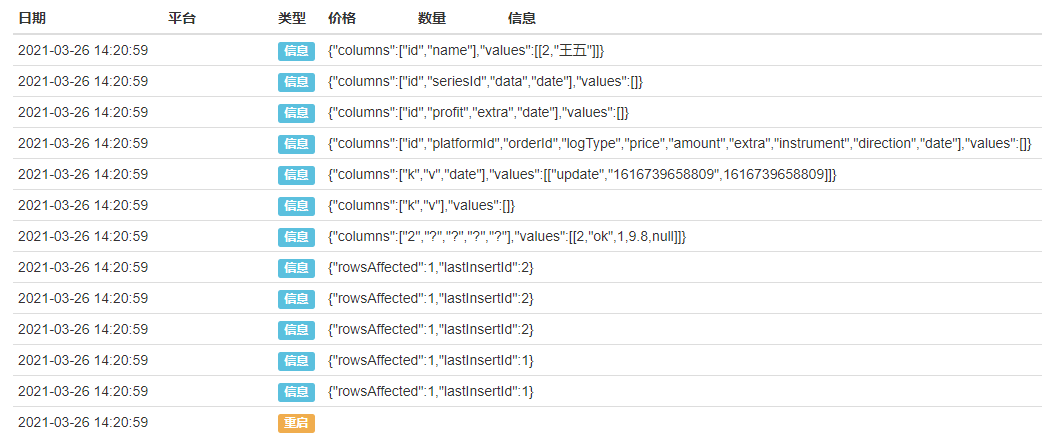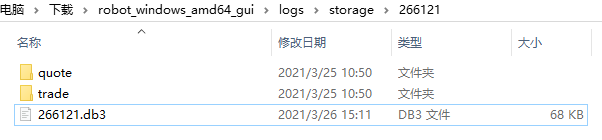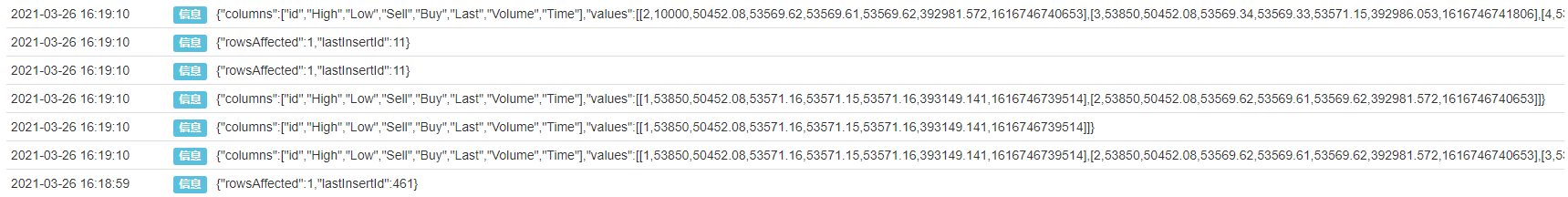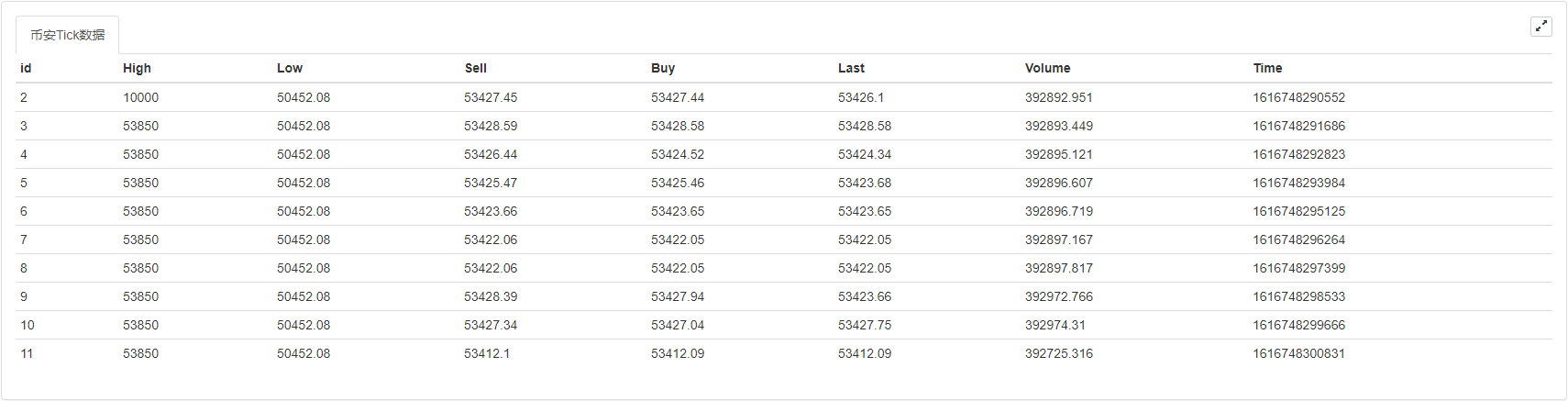# [原创] 利用SQLite构建发明者量化数据库

### 基础语法

SQLite的语法是不区分大小写的，不过有一些命令对大小写敏感，如GLOB和glob代表不同的含义。SQLite语句可以以任何关键字开始，如SELECT、INSERT、UPDATE、DELETE、ALTER、DROP等，它们分别表示：提取数据、插入数据、更新数据、删除数据、修改数据库、删除数据表。所有的语句以英文分号结束。下面是一个简单的数据库创建、增、删、改、查等操作：

``````function main() {
// 创建：如果“users”表不存在就创建一个，“id”是整数且自动增加，“name”是文本形式且不为空
Log(DBExec('CREATE TABLE IF NOT EXISTS "users" (id INTEGER PRIMARY KEY AUTOINCREMENT, name text not NULL);'));

// 增加：
Log(DBExec("INSERT INTO users(name) values('张三')"));
Log(DBExec("INSERT INTO users(name) values('李四')"));

// 删除：
Log(DBExec("DELETE FROM users WHERE id=1;"));

// 修改
Log(DBExec("UPDATE users SET name='王五' WHERE id=2"));

// 查询
Log(DBExec('select 2, ?, ?, ?, ?', 'ok', true,9.8,null));
Log(DBExec('select * from kvdb'));
Log(DBExec('select * from cfg'));
Log(DBExec('select * from log'));
Log(DBExec('select * from profit'));
Log(DBExec('select * from chart'));
Log(DBExec("selEct * from users"));
}
``````### 策略实例

``````function main() {
// 订阅合约
_C(exchange.SetContractType, 'swap');

// 创建数据表
DBExec('CREATE TABLE IF NOT EXISTS "tick" (id INTEGER PRIMARY KEY AUTOINCREMENT,'.concat(
'High FLOAT not NULL,',
'Low FLOAT not NULL,',
'Sell FLOAT not NULL,',
'Last FLOAT not NULL,',
'Volume INTEGER not NULL,',
'Time INTEGER not NULL);'
));

// 获取10个tick数据
while (true) {
let tick = exchange.GetTicker();
// 在tick表中增加数据
DBExec(`INSERT INTO tick(High, Low, Sell, Buy, Last, Volume, Time) values(\${tick.High}, \${tick.Low}, \${tick.Sell}, \${tick.Buy}, \${tick.Last}, \${tick.Volume}, \${tick.Time})`);
// 查询所有数据
let allDate = DBExec('select * from tick');
if (allDate.values.length > 10) {
break;
}
Sleep(1000);
}

// 查询所有数据
Log(DBExec('select * from tick'));

// 查询第一个数据
Log(DBExec('select * from tick limit 1'));

// 查询前两个数据
Log(DBExec('select * from tick limit 0,2'));

// 删除第一个数据
Log(DBExec('DELETE FROM tick WHERE id=1;'));

// 修改第二个数据
Log(DBExec('UPDATE tick SET High=10000 WHERE id=2'));

// 查询所有数据
let allDate = DBExec('select * from tick')
Log(allDate);
}
````````````    // 创建状态栏
let table = {
type: 'table',
title: '币安Tick数据',
cols: allDate.columns,
rows: allDate.values
}
LogStatus('`' + JSON.stringify(table) + '`');
``````### 完整策略代码

``````/*backtest
start: 2020-07-19 00:00:00
end: 2020-08-17 23:59:00
period: 15m
basePeriod: 15m
exchanges: [{"eid":"Binance","currency":"LTC_USDT"}]
*/

function main() {
Log(DBExec('DROP TABLE tick;'));
// 订阅合约
_C(exchange.SetContractType, 'swap');

// 创建数据表
DBExec('CREATE TABLE IF NOT EXISTS "tick" (id INTEGER PRIMARY KEY AUTOINCREMENT,'.concat(
'High FLOAT not NULL,',
'Low FLOAT not NULL,',
'Sell FLOAT not NULL,',
'Last FLOAT not NULL,',
'Volume INTEGER not NULL,',
'Time INTEGER not NULL);'
));

// 获取10个tick数据
while (true) {
let tick = exchange.GetTicker();
// 在tick表中增加数据
DBExec(`INSERT INTO tick(High, Low, Sell, Buy, Last, Volume, Time) values(\${tick.High}, \${tick.Low}, \${tick.Sell}, \${tick.Buy}, \${tick.Last}, \${tick.Volume}, \${tick.Time})`);
// 查询所有数据
let allDate = DBExec('select * from tick');
if (allDate.values.length > 10) {
break;
}
Sleep(1000);
}

// 查询所有数据
Log(DBExec('select * from tick'));

// 查询第一个数据
Log(DBExec('select * from tick limit 1'));

// 查询前两个数据
Log(DBExec('select * from tick limit 0,2'));

// 删除第一个数据
Log(DBExec('DELETE FROM tick WHERE id=1;'));

// 修改第二个数据
Log(DBExec('UPDATE tick SET High=10000 WHERE id=2'));

// 查询所有数据
let allDate = DBExec('select * from tick')
Log(allDate);

// 创建状态栏
let table = {
type: 'table',
title: '币安Tick数据',
cols: allDate.columns,
rows: allDate.values
}
LogStatus('`' + JSON.stringify(table) + '`');
}
``````

### 内存数据库

``````DBExec(":select 1,2,3");
``````